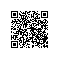# 一行jQuery代码打印九九乘法表

$(document).ready(function(){$("
").data('fact',8).css('margin','10px')
.appendTo(document.body)
.bind('error',function(){
$(this).parent().find("div:lt("+ ($(this).data('fact')) +")").eq(0)
.before($(this).clone(true).data('fact',$(this).data('fact') - 1))
.unbind('error')
.append(
$(" ").data('fact',$(this).data('fact')).width(20).height(20)
.css('display','inline').css('margin','10px')
.appendTo($(this)) .bind('focus',function(){$(this).text(($(this).data('fact') + 1) + "x" + ($(this).parent().data('fact') + 1) +
"=" +
($(this).data('fact') + 1)*($(this).parent().data('fact') + 1)
)
.parent().find("p:lt("+ ($(this).data('fact')) +")").eq(0) .before($(this).clone(true).data('fact',\$(this).data('fact') - 1))
.unbind('focus')
.parent().find("p").eq(0).triggerHandler('focus');
}).triggerHandler('focus')
).parent().find("div").eq(0).triggerHandler('error');
}).triggerHandler('error');
});使用钉钉扫一扫加入圈子
+ 订阅